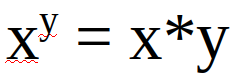# Mathematical sbi contest #4

in #puzzle3 years ago (edited)

Here you can keep your brain fit by solving math related problems and also earn SBI by doing so.
The problems usually contain a mathematical equation that in my opinion is fun to solve or has an interesting solution.
I will also only choose problems that can be solved without additional tools, so don't grab your calculator, you won't need it.
↓↑↓↑↓↑↓↑↓↑↓↑↓↑↓↑↓↑↓↑↓↑↓↑↓↑↓↑↓↑↓↑↓↑↓↑↓↑↓↑↓↑↓↑↓↑↓↑↓

# Rules

#### And one more thing: I only want integer solutions!

↓↑↓↑↓↑↓↑↓↑↓↑↓↑↓↑↓↑↓↑↓↑↓↑↓↑↓↑↓↑↓↑↓↑↓↑↓↑↓↑↓↑↓↑↓↑↓↑↓

# ProblemNo further restrictions today. The solutions are difficult enough to find.
↓↑↓↑↓↑↓↑↓↑↓↑↓↑↓↑↓↑↓↑↓↑↓↑↓↑↓↑↓↑↓↑↓↑↓↑↓↑↓↑↓↑↓↑↓↑↓↑↓

You can take a look at the results of the last contest here.

To everyone who already participated in a past contest, come back today and try a new problem(tell me if you don't want to be tagged):

In case no one gets a result(which I doubt), I will give away the 1 SBI to anyone who comments.

The contest is over. You can find the results here.

Sort:

x = 1, y = 2
or
x = 2, y = 1
or
x = 2, y = 2
or
x = 1, y = 1
or
wait, what was the question? I'll answer True!

No, this equation is not true for any values of x and y. If that were the case I wouldn't have chosen it.

By the way even your list of four pairs of x and y already contains a wrong pair. I suggest you rethink your answer.

Oh, right... x can't be 1, and y 2. Also forgot, x = 0, y = 1

The results can be found here.

x, y = 2

Posted using Partiko Android

The results can be found here.

3 years ago (edited)

-∞<x<∞; y=1
x=2; y=2

`∞<x<∞`? You know that is equivalent to "There is no possible value for x?"

3 years ago (edited)

Oh yeah I forgot a minus. I meant -∞<x<∞ just any integer x.

The results can be found here.

x=2,y=2
2*2=2 * 2